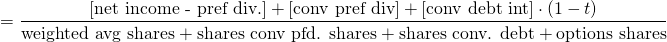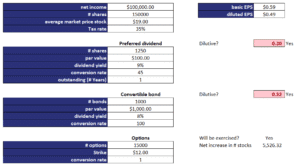# Diluted Earnings per Share

Diluted Earnings per Share (diluted EPS) is an earnings per share (EPS) measure that takes into account the possibility that stock options, convertible debt, or convertible preferred stock can be converted in to common equity. If exercised and converted to common stock, this will decrease the earnings per share.

To calculate earnings per share, we have to distinguish between dilutive securities and antidilutive securities:

• Dilutive securities are options, warrants, convertible debt, and convertible preferred stock that, when exercised, will decrease EPS
• Antidilutive securities are options, warrants, convertible debt, and convertible preferred stock that, when exercised, will increase EPS.

## Diluted EPS versus EPS

how to calculate diluted EPS? To learn about diluted EPS it is best to compare it to the regular EPS. In particular, diluted EPS is regular EPS but adjusted in a number of ways. Conceptually, the difference between basic and diluted EPS is that the former does not take into consideration that some securities may be converted into common stock, while the latter does.

First, let’s look at the numerator. The regular EPS’ numerator contains the income available to common shareholders, which is net income minus preferred dividends. In the case of dilutive securities, what happens is that:

• If convertible preferred stock is dilutive, convertible dividends are added back
• If convertible bonds are dilutive, we add the after-tax interest expense (interest times (1-tax rate)) of the bonds since we do not have to pay these when bonds are converted to stock

We also have to adjust the denominator. In particular, we have to add the number of common shares outstanding for the relevant period as if the dilutive securities are exercised. In other words, we have to calculate the number of fully diluted shares. If dilutive securities is issued during the year, we only need to consider the increase in the number of common shares for the part of the year that the shares were outstanding. Calculating the weighted average shares outstanding is the most tricky part in calculating diluted EPS.

Stock options and warrants are dilutive only when they are in-the-money, i.e. when exercising them is profitable. To determine the number of additional stocks that will be outstanding, we need to use the treasury stock method.

## Treasury stock method for options and warrants

The treasury stock method consists of three steps:

1. The funds received from the exercise of the options or warrants are used to buy outstanding stock at the average market price over the year
2. The net increase in number of stocks is equal to the total number of stocks created as a result of the exercise of the options or warrants minus the stocks bought using the proceeds received from the exercise.

## Diluted EPS calculation

The following diluted earnings per share formula should be used to calculate diluted EPSWhile the diluted EPS formula looks complicated, the intuition is pretty straight-forward. To the numerator we add back any income that would otherwise be distributed but that we do not have to distributed when the dilutive securities are exercised. In the denominator, we add the increase in common stock that results from the exercise of the dilutive securities.

It is very important to realize that we have to check, for each type of security, whether they are indeed dilutive. If not, we can ignore the security!

It is easy to perform the diluted earnings per share calculation using a spreadsheet. Below we include an Excel spreadsheet that implements an equity dilution calculator. The following figure illustrates the calculations needed.